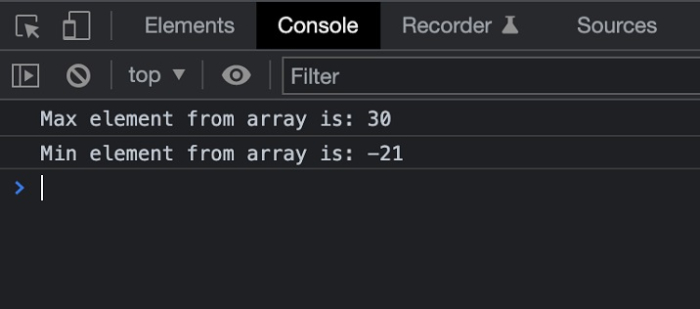# JavaScript: How to Find Min/Max Values Without Math Functions?

In this article, we will explore how to find the minimum and maximum values from an array without using the Math functions. Math functions including Math.min() and Math.max() returns the minimum and maximum values out of all the numbers passed in the array.

## Approach

We are going to use the same functionality of the Math functions that can be implemented using a loop.

This will iterate over the array elements using the for loop and will update the minimum and maximum element in a variable after comparing it with each element from the array.

On finding the value greater than the max value we will update the max variable and similarly for the min value.

## Example

In the below example, we find out the max and min values from the array without using the Math functions.

#Filename: index.html

<!DOCTYPE html>
<html lang="en">
<title>Find Min and Max</title>
<body>
<h1 style="color: green;">
Welcome to Tutorials Point
</h1>
<script>
// Defining the array to find out
// the min and max values
const array = [-21, 14, -19, 3, 30];

// Declaring the min and max value to
// save the minimum and maximum values

let max = array, min = array;
for (let i = 0; i < array.length; i++) {
// If the element is greater
// than the max value, replace max
if (array[i] > max) { max = array[i]; }

// If the element is lesser
// than the min value, replace min
if (array[i] < min) { min = array[i]; }
}
console.log("Max element from array is: " + max);
console.log("Min element from array is: " + min);
</script>
</body>
</html>

## Output

On successful execution of the above program, the browser will display the following result −

Welcome To Tutorials Point

And in the console, you will find the results, see the screenshot below −